# Fourier Series Calculator, On-line Application

### Basic Concepts and Principles

On-Line Fourier Series Calculator is an interactive app to calculate Fourier Series coefficients (Up to 10000 elements) for user-defined piecewise functions up to 5 pieces, for example.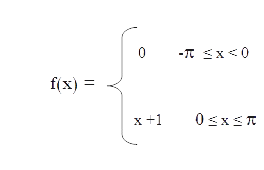Produces the result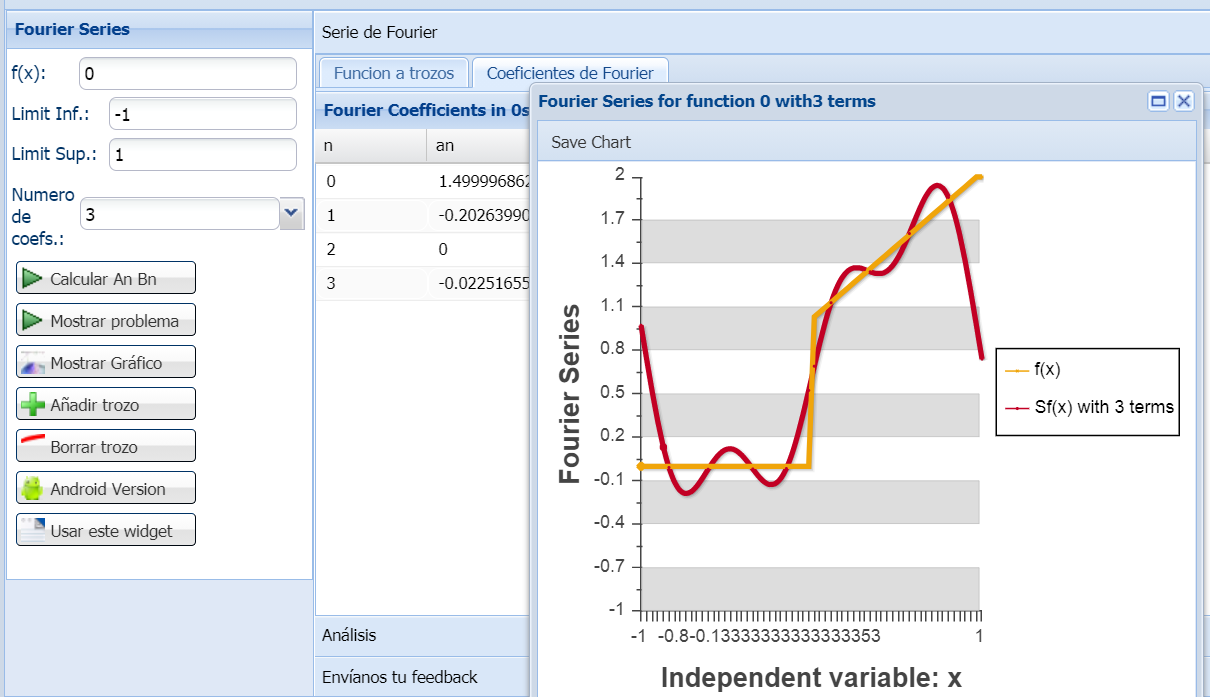Note that function must be in the integrable functions space or L1 on selected Interval as we shown at theory sections.

FourierSeries Calculator calculates Fourier Coefficients, analytic and numeric integrals and it is usefull to plot 1-variable functions and its Fourier series on a generic user-defined interval.

### Extended Theory

Calculations accuracy depends largely of size-interval introduzed and number of selected coefficients to calculate.
Use it is as follows.

1) Write the lower end of the range in the text box labeled Limit inf.
2) Enter the upper range in the text box labeled Limit Sup.
3) Write the function in the text box with the label function

In the piecewise function case, operate as follows
1) Write the lower end of the range in the text box labeled Limit inf.
2) Enter the upper range in the text box labeled Limit Sup.
3) Write the first function in the text box with the label function .
4) Enter the upper sub-range in the text box labeled Subinterval 1.
5) Write the function as defined in the first sub-interval in the text box labeled subinterval 1 .

For example suppose we have the piecewise functionThen the fields are filled as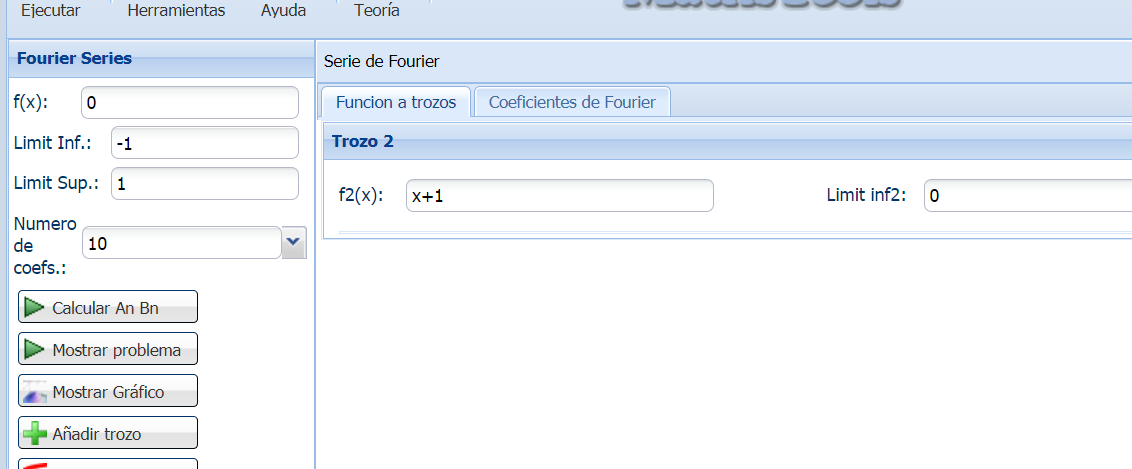After the An, Bn calculations, is possible to plot the function and its Fourier Series by clicking "Show Graph". At this case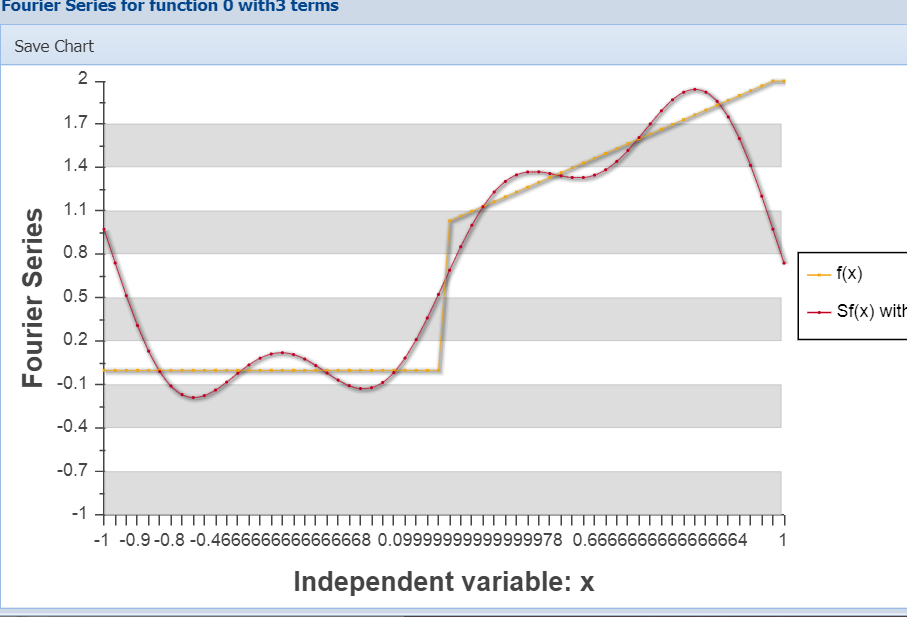By default, the problem begins with the continuous function and the interval followingHow it works?
To calculate Fourier coefficients integration methods seen in the numerical methods section are applied. With which we can approximate the integralsIn the case of Fourier coefficients, there are several methods to make the calculations, being exposed here created by the owners of Mathstools .
To calculate the derivative of the function: uses severeal numerical methods to dereivate.
To calculate the primitive function: numerical integration methods seen in the numerical methods section are applied.
Note that in numerical analysis, errors are obtained due to the particular methods and the limits of computer arithmetic.
In the the Fourier coefficients calculations case, it depends on the function and size of the chosen integration interval. In default probelm the error in calculating the Fourier coefficients is O (1e-8). For the numerical integration is O (1e-11) and in the derivative it is O (1e-14).

# Was useful? want add anything?

Post here

### Carlos:

2020-01-17 18:10
Hi, Rao

Thanks for your comment, I'd procced as follows:

Because you are interested in period T=2 i will consider the interval [0,2] and only a piece.

I did'n understood your funcion, so i write two cases (i use x instead t as independent variable):

CASE 1: if your function is f(x) = -3/(2*x) +2 such function has not Fourier Series.
CASE 2: if your function is f(x) = -3*x/2 + 2 such function has not Fourier Series.

In case2 i proceed as follows: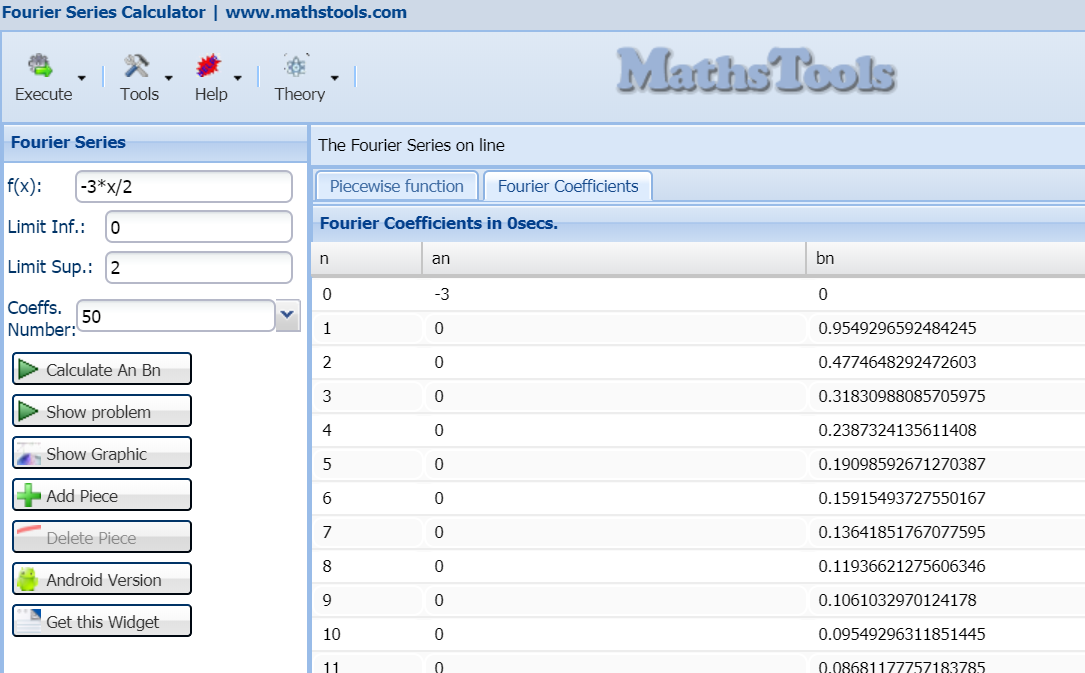Note that, i' ve used only a piece into interval [0,2] as said

Regards
Carlos

### Xander:

2013-03-25 20:11:11
It would be really nice to be able to see the steps of how it calculated the fourier co-efficients.

Post here